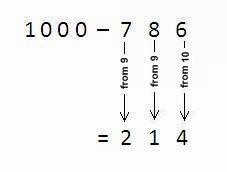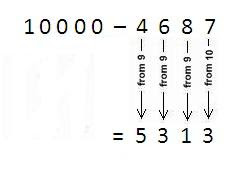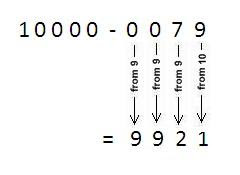# How to do Fast Subtraction - Vedic Mathematics Method

Vedic mathematics is an ancient method of Indian mathematics which was rediscovered from the Vedas between 1911 and 1918 by Sri Bharati Krishna Tirthaji (1884-1960).

In this section I am going to post vedic mathematics tricks daily, which will help you to make calculations easier and faster than normal methods. So let us start our Vedic maths first tutorial.

In this tutorial we are going to make subtraction of any number from 100, 1000, 1000, 10000 and so on.

## How to do fast subtraction within seconds

Subtract all digits from 9 and last digit from 10 (See example).

Example 1: Subtract 786 from 1000.

[caption id="" align="aligncenter" width="227"]Vedic maths subtraction[/caption]

Step: In this example we have subtracted 7 and 8 from 9 and then 6 from 10 resulting 214.

Example 2: Subtract 4687 from 10000Step: Here 4, 6 and 8 are subtracted from 9 and then 7 is subtracted from 10.

Important note: When we have more zeros than figures in the number being subtracted then don’t forget to write zeros before the number so as to make same length (See example).

Example 3: Subtract 79 from 10000.

Solution: Here we have 2 digits in 79 and 4 zeros in 10000. Therefore write 0079.### You may also like

1.